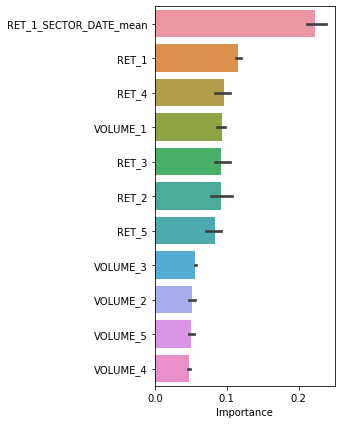# Benchmark QRT¶

This notebook illustrates a simple benchmark example that should help novice participants to start the competition.

## Used libraries¶

In :
import seaborn as sns
import numpy as np
import pandas as pd
from sklearn.ensemble import RandomForestClassifier
from sklearn.metrics import accuracy_score
from sklearn.model_selection import KFold


The train and test inputs are composed of 46 features.

The target of this challenge is RET and corresponds to the fact that the return is in the top 50% of highest stock returns.

Since the median is very close to 0, this information should not change much with the idea to predict the sign of the return.

In :
x_train = pd.read_csv('./x_train.csv', index_col='ID')
train = pd.concat([x_train, y_train], axis=1)

Out:
DATE STOCK INDUSTRY INDUSTRY_GROUP SECTOR SUB_INDUSTRY RET_1 VOLUME_1 RET_2 VOLUME_2 ... VOLUME_16 RET_17 VOLUME_17 RET_18 VOLUME_18 RET_19 VOLUME_19 RET_20 VOLUME_20 RET
ID
0 0 2 18 5 3 44 -0.015748 0.147931 -0.015504 0.179183 ... 0.630899 0.003254 -0.379412 0.008752 -0.110597 -0.012959 0.174521 -0.002155 -0.000937 True
1 0 3 43 15 6 104 0.003984 NaN -0.090580 NaN ... NaN 0.003774 NaN -0.018518 NaN -0.028777 NaN -0.034722 NaN True
2 0 4 57 20 8 142 0.000440 -0.096282 -0.058896 0.084771 ... -0.010336 -0.017612 -0.354333 -0.006562 -0.519391 -0.012101 -0.356157 -0.006867 -0.308868 False
3 0 8 1 1 1 2 0.031298 -0.429540 0.007756 -0.089919 ... 0.012105 0.033824 -0.290178 -0.001468 -0.663834 -0.013520 -0.562126 -0.036745 -0.631458 False
4 0 14 36 12 5 92 0.027273 -0.847155 -0.039302 -0.943033 ... -0.277083 -0.012659 0.139086 0.004237 -0.017547 0.004256 0.579510 -0.040817 0.802806 False

5 rows × 47 columns

## Feature Engineering¶

The main drawback in this challenge would be to deal with the noise. To do that, we could create some feature that aggregate features with some statistics.

The following cell computes statistics on a given target conditionally to some features. For example, we want to generate a feature that describe the mean of RET_1 conditionally to the SECTOR and the DATE.

Ideas of improvement: change shifts, the conditional features, the statistics, and the target.

In :
# Feature engineering
new_features = []

# Conditional aggregated features
shifts =   # Choose some different shifts
statistics = ['mean']  # the type of stat
gb_features = ['SECTOR', 'DATE']
target_feature = 'RET'
tmp_name = '_'.join(gb_features)
for shift in shifts:
for stat in statistics:
name = f'{target_feature}_{shift}_{tmp_name}_{stat}'
feat = f'{target_feature}_{shift}'
new_features.append(name)
for data in [train, test]:
data[name] = data.groupby(gb_features)[feat].transform(stat)


## Feature selection¶

To reduce the number of feature (and the noise) we only consider the 5 last days of RET and VOLUME in addition to the newly created feature.

In :
target = 'RET'

n_shifts = 5  # If you don't want all the shifts to reduce noise
features = ['RET_%d' % (i + 1) for i in range(n_shifts)]
features += ['VOLUME_%d' % (i + 1) for i in range(n_shifts)]
features += new_features  # The conditional features

Out:
RET_1 RET_2 RET_3 RET_4 RET_5 VOLUME_1 VOLUME_2 VOLUME_3 VOLUME_4 VOLUME_5 RET_1_SECTOR_DATE_mean
ID
0 -0.015748 -0.015504 0.010972 -0.014672 0.016483 0.147931 0.179183 0.033832 -0.362868 -0.972920 0.009178
1 0.003984 -0.090580 0.018826 -0.025540 -0.038062 NaN NaN NaN NaN NaN 0.006477
2 0.000440 -0.058896 -0.009042 0.024852 0.009354 -0.096282 0.084771 -0.298777 -0.157421 0.091455 0.013449
3 0.031298 0.007756 -0.004632 -0.019677 0.003544 -0.429540 -0.089919 -0.639737 -0.940163 -0.882464 0.017253
4 0.027273 -0.039302 0.000000 0.000000 0.022321 -0.847155 -0.943033 -1.180629 -1.313896 -1.204398 0.006241

## Model and local score¶

A Random Forest (RF) model is chosen for the Benchmark. We consider a large number of tree with a quiet small depth. The missing values are simply filled with 0. A KFold is done on the dates (using DATE) for a local scoring of the model.

Ideas of improvements: Tune the RF hyperparameters, deal with the missing values, change the features, consider another model, ...

In :
X_train = train[features]
y_train = train[target]

# A quiet large number of trees with low depth to prevent overfits
rf_params = {
'n_estimators': 500,
'max_depth': 2**3,
'random_state': 0,
'n_jobs': -1
}

train_dates = train['DATE'].unique()
test_dates = test['DATE'].unique()

n_splits = 4
scores = []
models = []

splits = KFold(n_splits=n_splits, random_state=0,
shuffle=True).split(train_dates)

for i, (local_train_dates_ids, local_test_dates_ids) in enumerate(splits):
local_train_dates = train_dates[local_train_dates_ids]
local_test_dates = train_dates[local_test_dates_ids]

local_train_ids = train['DATE'].isin(local_train_dates)
local_test_ids = train['DATE'].isin(local_test_dates)

X_local_train = X_train.loc[local_train_ids]
y_local_train = y_train.loc[local_train_ids]
X_local_test = X_train.loc[local_test_ids]
y_local_test = y_train.loc[local_test_ids]

X_local_train = X_local_train.fillna(0)
X_local_test = X_local_test.fillna(0)

model = RandomForestClassifier(**rf_params)
model.fit(X_local_train, y_local_train)

y_local_pred = model.predict_proba(X_local_test)[:, 1]

sub = train.loc[local_test_ids].copy()
sub['pred'] = y_local_pred
y_local_pred = sub.groupby('DATE')['pred'].transform(lambda x: x > x.median()).values

models.append(model)
score = accuracy_score(y_local_test, y_local_pred)
scores.append(score)
print(f"Fold {i+1} - Accuracy: {score* 100:.2f}%")

mean = np.mean(scores)*100
std = np.std(scores)*100
u = (mean + std)
l = (mean - std)
print(f'Accuracy: {mean:.2f}% [{l:.2f} ; {u:.2f}] (+- {std:.2f})')

Fold 1 - Accuracy: 51.87%
Fold 2 - Accuracy: 50.65%
Fold 3 - Accuracy: 51.11%
Fold 4 - Accuracy: 52.10%
Accuracy: 51.43% [50.85 ; 52.02] (+- 0.58)

In :
feature_importances = pd.DataFrame([model.feature_importances_ for model in models], columns=features)
import matplotlib.pyplot as plt

fig, ax = plt.subplots(figsize=(5, 6))
sns.barplot(data=feature_importances, orient='h', order=feature_importances.mean().sort_values(ascending=False).index, ax=ax)
ax.set_xlabel('Importance')
fig.tight_layout()
fig.savefig('./feature_importance_rf.pdf')## Generate the submission¶

The same parameters of the RF model are considered. With that we build a new RF model on the entire train dataset. The predictions are saved in a .csv file.

In :
X_test = test[features]

rf_params['random_state'] = 0
model = RandomForestClassifier(**rf_params)
model.fit(X_train.fillna(0), y_train)
y_pred = model.predict_proba(X_test.fillna(0))[:, 1]

sub = test.copy()
sub['pred'] = y_pred
y_pred = sub.groupby('DATE')['pred'].transform(
lambda x: x > x.median()).values

submission = pd.Series(y_pred)
submission.index = test.index
submission.name = target